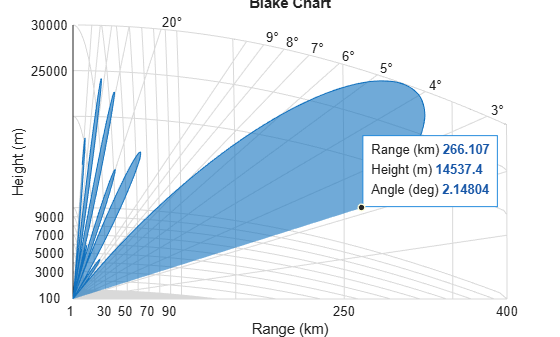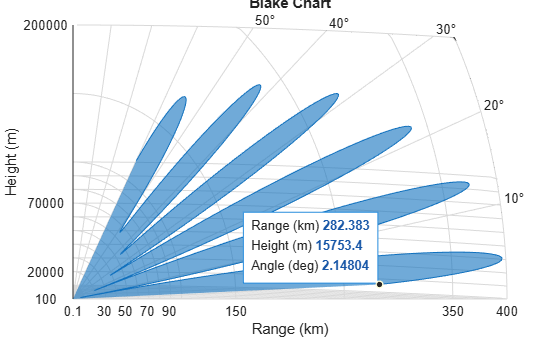Main Content

# radarvcd

Vertical coverage diagram

## Syntax

``````[vcp,vcpangles] = radarvcd(freq,rfs,anht)``````
``````[vcp,vcpangles] = radarvcd(___,Name,Value)``````
``radarvcd(___)``

## Description

example

``````[vcp,vcpangles] = radarvcd(freq,rfs,anht)``` calculates the vertical coverage pattern of a narrowband radar antenna. The Vertical Coverage Pattern is the radar’s range, `vcp`, as a function of elevation angle, `vcpangles`. The vertical coverage pattern depends upon three parameters. These parameters are the radar’s maximum free-space detection range, `rfs`, the radar frequency, `freq`, and the antenna height, `anht`.```

example

``````[vcp,vcpangles] = radarvcd(___,Name,Value)``` allows you to specify additional input parameters as Name-Value pairs. You can specify additional name-value pair arguments in any order as (`Name1`,`Value1`,...,`NameN`,`ValueN`). This syntax can use any of the input arguments in the previous syntax.```

example

````radarvcd(___)` displays the vertical coverage diagram for a radar system. The plot is the locus of points of maximum radar range as a function of target elevation. This plot is also known as the Blake chart. To create this chart, `radarvcd` invokes the function `blakechart` using default parameters. To produce a Blake chart with different parameters, first call `radarvcd` to obtain `vcp` and `vcpangles`. Then, call `blakechart` with user-specified parameters. This syntax can use any of the input arguments in the previous syntaxes.```

## Examples

collapse all

Set the frequency to 100 MHz, the antenna height to 10 m, and the free-space range to 200 km. The antenna pattern, surface roughness, antenna tilt angle, and field polarization assume their default values as specified in the `AntennaPattern`, `SurfaceRoughness`, `TiltAngle`, and `Polarization` properties.

Obtain an array of vertical coverage pattern values and angles.

```freq = 100e6; ant_height = 10; rng_fs = 200; [vcp,vcpangles] = radarvcd(freq,rng_fs,ant_height);```

To see the vertical coverage pattern, omit the output arguments.

```freq = 100e6; ant_height = 10; rng_fs = 200; radarvcd(freq,rng_fs,ant_height);```Set the frequency to 100 MHz, the antenna height to 10 m, and the free-space range to 200 km. The antenna pattern is a sinc function with 45° half-power width. The surface roughness is set to 1 m. The antenna tilt angle is set to 0°, and the field polarization is horizontal.

```pat_angles = linspace(-90,90,361)'; pat_u = 1.39157/sind(45/2)*sind(pat_angles); pat = sinc(pat_u/pi); freq = 100e6; ant_height = 10; rng_fs = 200; tilt_ang = 0; [vcp,vcpangles] = radarvcd(freq,rng_fs,ant_height,... 'RangeUnit','km','HeightUnit','m',... 'AntennaPattern',pat,... 'PatternAngles',pat_angles,... 'TiltAngle',tilt_ang,'SurfaceRoughness',1);```

Plot the range-height-angle curve (Blake chart) for a radar with a user-specified antenna pattern.

Define a sinc-function antenna pattern with a half-power beamwidth of 90 degrees.

```pat_angles = linspace(-90,90,361)'; pat_u = 1.39157/sind(90/2)*sind(pat_angles); pat = sinc(pat_u/pi);```

Specify a radar that transmits at 100 MHz. The free-space range is 200 km, the antenna height is 10 meters, the antenna tilt angle is zero degrees, and the surface roughness is one meter.

```freq = 100e6; ant_height = 10; rng_fs = 200; tilt_ang = 0; surf_roughness = 1;```

Create the radar range-height-angle plot.

```radarvcd(freq,rng_fs,ant_height,... 'RangeUnit','km','HeightUnit','m',... 'AntennaPattern',pat,... 'PatternAngles',pat_angles,... 'TiltAngle',tilt_ang,... 'SurfaceRoughness',surf_roughness);```## Input Arguments

collapse all

Radar frequency specified as a real-valued scalar less than 10 GHz (10e9).

Example: 100e6

Data Types: `double`

Free-space range specified as a real-valued scalar. Range units are set by the `RangeUnit` Name-Value pair.

Example: 100e3

Data Types: `double`

Radar antenna height specified as a real-valued scalar. Height units are set by the `HeightUnit` Name-Value pair.

Example: 10

Data Types: `double`

### Name-Value Pair Arguments

Specify optional comma-separated pairs of `Name,Value` arguments. `Name` is the argument name and `Value` is the corresponding value. `Name` must appear inside quotes. You can specify several name and value pair arguments in any order as `Name1,Value1,...,NameN,ValueN`.

Example: ‘HeightUnit’, k‘m’

Radar range units denoting kilometers, nautical miles, miles, feet or meters. This name-value pair specifies the units for the free-space range argument, `rfs`, and the output vertical coverage pattern, `vcp`.

Example: `'mi'`

Data Types: `char`

Antenna height units denoting meters, nautical miles, miles, kilometers, or feet. This name-value pair specifies the units for the antenna height, `anht`, and the `'SurfaceRoughness'` name-value pair.

Example: `'m'`

Data Types: `char`

Transmitted wave polarization specified as `'H'` for horizontal polarization and `'V'` for vertical polarization.

Example: `'V'`

Data Types: `char`

Dielectric constant of reflecting surface specified as complex-valued scalar. When omitted, the dielectric constant is taken from a frequency-dependent seawater dielectric model derived in Blake.

Example: 70

Data Types: `double`

Surface roughness specified as a non-negative real scalar. Surface roughness is a measure of the height variation of the reflecting surface. The roughness is modeled as a sinusoid wave with crest-to-trough height given by this value. A value of 0 indicates a smooth surface. The units for surface roughness height is specified by the value of the `'HeightUnit'` Name-Value pair.

Example: 2

Data Types: `double`

Antenna elevation pattern, specified as a real-valued N-by-1 column vector. Values for `'AntennaPattern'` must be specified together with values for `'PatternAngles'`.

Example: `cosd([–90:90])`

Data Types: `double`

Antenna pattern elevation angles specified as a real-valued N-by-1 column vector. The size of the vector specified by `'PatternAngles'` must be the same as that specified by `'AntennaPattern'`. Angle units are expressed in degrees and must lie between –90° and 90°. In general, to properly compute the coverage, the antenna pattern should fill the whole range from –90° to 90°.

Example: `[-90:90]`

Data Types: `double`

Antenna tilt angle specified as a real-valued scalar. The tilt angle is the elevation angle of the antenna with respect to the surface. Angle units are expressed in degrees.

Example: 10

Data Types: `double`

Maximum elevation angle, specified as a real-valued scalar. The maximum elevation angle is the largest angle for which the vertical coverage pattern is calculated. Angle units are expressed in degrees.

Example: 70

Data Types: `double`

## Output Arguments

collapse all

Vertical coverage pattern returned as a real-valued, K-by-1 column vector. The vertical coverage pattern is the actual maximum range of the radar. Each entry of the vertical coverage pattern corresponds to one of the angles returned in `vcpangles`.

Vertical coverage pattern angles returned as a K-by-1 column vector. The angles range from –90° to 90°.

## More About

collapse all

### Vertical Coverage Pattern

The maximum detection range of a radar antenna can differ, depending on placement. Suppose you place a radar antenna near a reflecting surface, such as the earth's land or sea surface and computed maximum detection range. If you then move the same radar antenna to free space far from any boundaries, a different maximum detection range would result. This is an effect of multi-path interference that occurs when waves, reflected from the surface, constructively add to or nullify the direct path signal from the radar to a target. Multipath interference gives rise to a series of lobes in the vertical plane. The vertical coverage pattern is the plot of the actual maximum detection range of the radar versus target elevation and depends upon the maximum free-space detection range and target elevation angle. See Blake .

 Blake, L.V. Machine Plotting of Radar Vertical-Plane Coverage Diagrams. Naval Research Laboratory Report 7098, 1970.

## SupportGet trial now